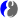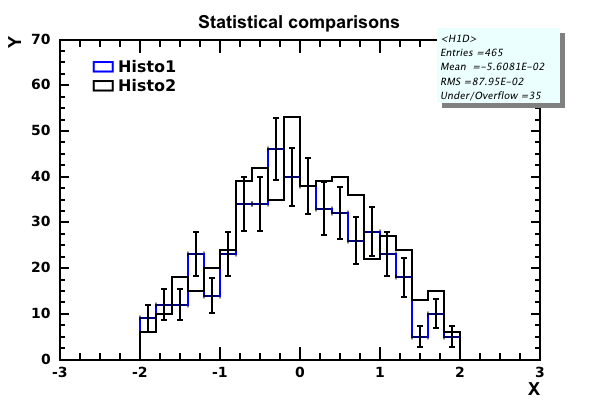# DMelt:Statistics/3 Statistical Tests

(diff) ← Older revision | Latest revision (diff) | Newer revision → (diff)
Limitted access. First login to DataMelt if you are a full DataMelt member. Then login to HandWiki as a user.

# Statistical tests

DataMelt can be used to perform various types of Statistical_hypothesis_testing. For example, let us run a simplePearson's chi-squared test on data comparing data and a function. We will use 3-data points (with statistical uncertainties) and a function $\displaystyle{ 10+x^3 }$. Here we calculate Pearson's_chi-squared_test and evaluated the p-value (probability that data agree with analytic function given in this example).

from jhplot  import *

p=P1D("Data")
f=F1D('10+x*x*x',1,3)
print p.compareChi2(f)
c=HPlot()
c.visible()
c.setAutoRange()
c.draw(p);  c.draw(f)

The output of this code, in addition to the image shown above, is this line:

{chi2: 0.8322222222222222, ndf: 3.0, p-value: 0.8417453843895332}

## Histogram tests

When using DataMelt, two distributions (1D and 2D histograms, P1D data points) can be compared by applying several statistical tests. The following statistical comparisons are available

Below we will look into this in more details. Consider a simple statistical test: compare 2 histograms. You can generate 2 similar histograms using this code snippet:

from java.awt import Color
from java.util import Random
from jhplot  import *

c1 = HPlotJa("Canvas")
c1.setGTitle("Statistical comparisons")
c1.setAutoRange()
c1.visible()

h1 = H1D("Histo1",20, -2, 2.0)
h1.setColor(Color.blue)
h2 = H1D("Histo2",20, -2, 2.0)
r = Random()
for i in range(500):
h1.fill(r.nextGaussian())
h2.fill(r.nextGaussian())
if (i<100): h2.fill(2*r.nextGaussian()+2)

h1.setErrAll(1)
h2.setErrAll(0)
c1.draw(h1)
c1.draw(h2)

from hep.aida.util.comparison import *
# Compare the two histograms with the "AndersonDarling" algorithm
result = StatisticalComparison.compare(h1.get(), h2.get(),"AndersonDarling","")
print    "AndersonDarling method=",result.quality(),"/",result.nDof()

# Compare the two histograms with the "Chi2" algorithm with rejection level at 1%.
result = StatisticalComparison.compare(h1.get(), h2.get(),"chi2","rejectionLevel=0.01")
print    "Chi2 method=",result.quality() ,"/",result.nDof()

# Goodman","KolmogorovSmirnovChi2Approx","KSChi2Approx
result = StatisticalComparison.compare(h1.get(), h2.get(),"Goodman","rejectionLevel=0.01")
print    "Goodman method=",result.quality() ,"/",result.nDof()

# KolmogorovSmirnov
result = StatisticalComparison.compare(h1.get(), h2.get(),"KolmogorovSmirnov","rejectionLevel=0.01")
print    "KolmogorovSmirnov  method=",result.quality() ,"/",result.nDof()

# Kuiper: works for P1D
# result = StatisticalComparison.compare(h1.get(), h2.get(),"Kuiper","rejectionLevel=0.01")
# print    "Kuiper  method=",result.quality() ,"/",result.nDof()

Here we show statistical uncertainties only for the first (blue) histogram (see the method setErrAll(0)). The output of this code is shown belowNow we can perform a several tests to calculate the degree of similarity of these distributions (including their uncertainties). Below we show a code which compares these two histograms and calculate Chi2 per degree of freedom:

from java.awt import Color
from java.util import Random
from jhplot  import *

c1 = HPlotJa("Canvas")
c1.setGTitle("Statistical comparisons")
c1.setAutoRange()
c1.visible()

h1 = H1D("Histo1",20, -2, 2.0)
h1.setColor(Color.blue)
h2 = H1D("Histo2",20, -2, 2.0)
r = Random()
for i in range(500):
h1.fill(r.nextGaussian())
h2.fill(r.nextGaussian())
if (i<100): h2.fill(2*r.nextGaussian()+2)

h1.setErrAll(1)
h2.setErrAll(0)
c1.draw(h1)
c1.draw(h2)

from hep.aida.util.comparison import *
# Compare the two histograms with the "AndersonDarling" algorithm
result = StatisticalComparison.compare(h1.get(), h2.get(),"AndersonDarling","")
print    "AndersonDarling method=",result.quality(),"/",result.nDof()

# Compare the two histograms with the "Chi2" algorithm with rejection level at 1%.
result = StatisticalComparison.compare(h1.get(), h2.get(),"chi2","rejectionLevel=0.01")
print    "Chi2 method=",result.quality() ,"/",result.nDof()

# Goodman","KolmogorovSmirnovChi2Approx","KSChi2Approx
result = StatisticalComparison.compare(h1.get(), h2.get(),"Goodman","rejectionLevel=0.01")
print    "Goodman method=",result.quality() ,"/",result.nDof()

# KolmogorovSmirnov
result = StatisticalComparison.compare(h1.get(), h2.get(),"KolmogorovSmirnov","rejectionLevel=0.01")
print    "KolmogorovSmirnov  method=",result.quality() ,"/",result.nDof()

# Kuiper: works for P1D
# result = StatisticalComparison.compare(h1.get(), h2.get(),"Kuiper","rejectionLevel=0.01")
# print    "Kuiper  method=",result.quality() ,"/",result.nDof()

The output of this script is shown here:

AndersonDarling method= 2.21779532164 / 20
Chi2 method= 0.786556311893 / 20
Goodman method= 0.624205522632 / 20
KolmogorovSmirnov  method= 0.419524135727 / 20

## Non-parametric tests

This section contains a description of many non-parametric tests that were included using third-party libraries. In particular, we will show easy scripting using the JavaNPST library that is included in DataMelt and interfaced with Java scripting languages. The library contains

• Tests of goodness (Chi-Square, Kolmogorov-Smirnov, Lilliefors, Anderson-Darling),
• Tests of randomness (Number of Runs, Von Neumann, Runs Up and Down)
• One-sample and paired-samples (Confidence Quantile, Population Quantile, Wilcoxon Signed-Ranks )
• Two-Sample general procedures (Wald-Wolfowitz, Control Median, Kolmogorov-Smirnov)
• Scale problem (David-Barton , Klotz , Freund-Ansari-Bradley , David-Barton
• Equality of independent samples (Extended Median test , Jonckheere-Terpstra, Charkraborti-Desu)
• Association in multiple classifications (Friedman, Concordance Coefficient, Incomplete Concordance, Partial Correlation )
• Analysis of count data (Contingency Coefficient, Fisher's exact test, Multinomial Equality, Ordered Equality)

You can view Java API for all these statistical tests using this linkStatistical tests API. On this page, select the needed method listened in the section "Direct Known Subclasses:".

To be more specific, let us consider a few practical examples. Let us consider a simple Jython script that tests randomness of a numeric sequence using the Von Neumann test.

Let us perform Von Neumann test for a sequence of numbers

12362,12439,12057,13955,14123,3698,16523,18610,1442,20310,21500,23000,21316

The result of this test is shown here:

Results of Number of Runs test:
''''''''''''''''''''''''''''''''''''''''''''''''''''''''''''
Von Neumann test (ranks test of randomness)
''''''''''''''''''''''''''''''''''''''''''''''''''''''''''''
NM statistic: 231
RVN statistic: 1.269231
Exact P-Value (Left tail, Too few runs): 0.1
Exact P-Value (Right tail, Too many runs): 1
Exact P-Value (Double tail, Non randomness): 0.2
Asymptotic P-Value (Left tail, Too few runs): 0.080571
Asymptotic P-Value (Right tail, Too many runs): 0.919429
Asymptotic P-Value (Double tail, Non randomness): 0.161142

which is performed with this simple code:

from javanpst.tests.randomness.vonNeumannTest import VonNeumannTest
from javanpst.data.structures.sequence import NumericSequence

d = NumericSequence([12362,12439,12057,13955,14123,3698,16523,18610,1442,20310,21500,23000,21316])
t1 = VonNeumannTest(d)
t1.doTest()
print "Results of VonNeumann  test:"+t1.printReport()

Let us consider another example. This time we will perform the Friedman test:

The input for this test will be the matrix:

[[12,23,33],[23,23,11],[23,11,23]]

The output of our test is shown below:

'''''''''''''''''''''''''''
Friedman test
'''''''''''''''''''''''''''
Sum of ranks:
S1	S2	S3
6	5.5	6.5
Average ranks:
S1	S2	S3
2	1.833333	2.166667
S statistic: 0.5
Q statistic: 0.2
P-Value computed :0.904837

The code for this example is given below: